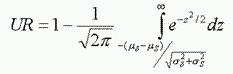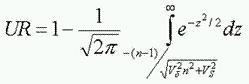# Stress/Strength

Stress/Strength analysis method determines the probability of failure based on the probability of stress exceeding strength.
Calculation of Failure Probability (Unreliability) by:
• Distributions of Stress and Strength
• Variation information between Stress and Strength (Factor of Safety n and Variations)

Having distributions of Stress and Strength, we may calculate failure probability (Unreliability):Having variation information between stress and strength (Factor of Safety n and Variations), we may also calculate the unreliability: